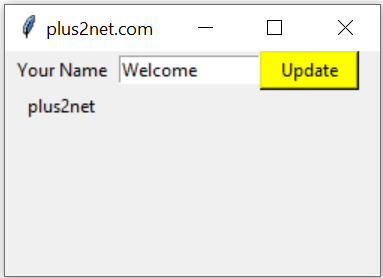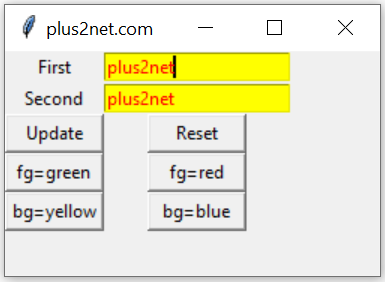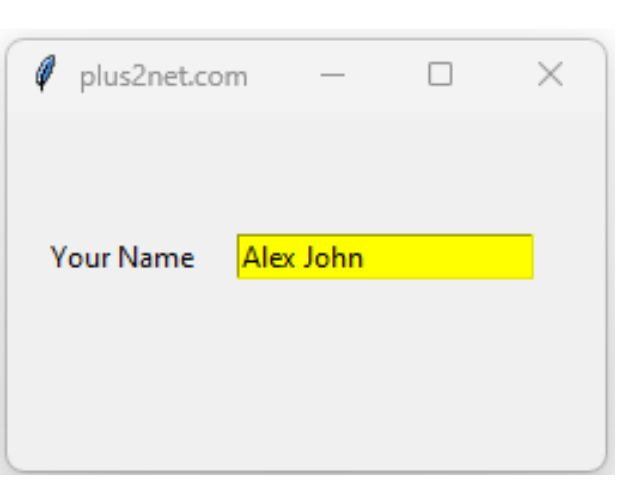# Python tkinter Entry

We will keep one Label along with a Entry box.
User input for single line of text. ( for multi line of text use Text)
``t1=tk.Entry(parent_window,options)``
`parent_window` : Declared Parent window
`options` : Various options can be added, list is given below.``````import tkinter as tk
my_w = tk.Tk()
my_w.geometry("250x150")

l1.grid(row=1,column=1)

e1 = tk.Entry(my_w,   width=20,bg='yellow') # added one Entry box
e1.grid(row=1,column=2)

my_w.mainloop()``````
Tkinter entry widget with options and methods to manage user inputs. Reading & setting default data

## Options

 `bg` Color of the background. We can use Hex value or colour name. ( bg='green' or bg='#ffff00') `bd` Border size, default value is 2 `cursor` Shape of the cursor while moving over the Entry widget. A list of shapes are available at Button Page. `font` font style ,size used. Check the example below `fg` font colour. Check the example below `justify` Alignment of text. Values are 'left','center' or 'right' `relief` The borders 3 D effect style. It can take these values 'raised' , 'sunken' ,'flat', 'ridge', 'solid' & 'groove' `selectbackground` Colour of the background when text is selected `selectforeground` Colour of the font when text is selected``selectbackground='#ffff00',selectforeground='#0000ff'`` `selectborderwidth` Size of the border around the selection, default value is 0 `show` The entries can be masked, used when entering passwords. Check the example below. `state` Values can be 'disabled', 'normal','readonly'. Check the example below. `textvariable` variable associated with the Entry. Used for setting or reading the user entered data. Usually StringVar is used. `width` width of the widget, default value is 20 `xscrollcommand` Connecting Entry widget to a scrollbar

## Reading data of Entry box and entering data

We can read the data entered inside the Entry text box ( e1 is the Entry box )
``e1.get()``

## To Delete text of Entry box

To clear the text available inside Entry box use this.
``e1.delete(0,END)``

## Using a Reset button

We can use one click event of a button to clear or Reset the text entered in an Entry box.
``````import tkinter  as tk
my_w = tk.Tk()
my_w.geometry("380x200")

e1=tk.Entry(my_w,text='plus2net',font=24)

b1=tk.Button(my_w,text='Reset',font=24,command=lambda:e1.delete(0,'end'))
my_w.mainloop()``````

## Resetting all Entry boxes

Irrespective of number of Entry boxes we used in our application, we can remove all user entered data by using winfo_children() which returns all the widget classes of the Tkinter window. Here is the code
``````for widget in my_w.winfo_children():
if isinstance(widget, tk.Entry):  # If this is an Entry widget
widget.delete(0,'end') # Delete all entries ``````

## Default text in Entry widget

We can keep any default text or data from any other input system inside an Entry box. For this we will use one StringVar().

We can bind the StringVar() e1_str to our Entry box e1. Now we are adding one default text Welcome to the Entry text.
``````e1_str=tk.StringVar(my_w) # Declaring String variable
e1 = tk.Entry(my_w,textvariable=e1_str,width=15) # added one Entry box
e1.grid(row=1,column=2)
e1_str.set('Welcome')``````
Using the above methods we will create one application where on Click of a button the data entered by the user in Entry box is displayed and default text is changed to Welcome.``````def my_upd():
my_str.set(e1.get()) # read and assign text to StringVar()
e1_str.set('Welcome')# Update the Entry box text``````
Here on Click of the button the function my_upd() is executed. Inside this function we will first read the text entered by user and assign the same to another Label ( l2 ) .

In next line we will assign the string 'Welcome' to the StringVar() binded to Entry box ( e1 ).
``````import tkinter as tk
my_w = tk.Tk()
my_w.geometry("250x150")

l1.grid(row=1,column=1)
e1_str=tk.StringVar()
e1 = tk.Entry(my_w,textvariable=e1_str,width=15) # added one Entry box
e1.grid(row=1,column=2)

b1 = tk.Button(my_w, text='Update', width=8,bg='yellow',
command=lambda: my_upd())
b1.grid(row=1,column=3)

my_str = tk.StringVar()
l2 = tk.Label(my_w,  text='Output',textvariable=my_str, width=10 )
l2.grid(row=2,column=1)

def my_upd():
my_str.set(e1.get()) # read and assign text to StringVar()
e1_str.set('Welcome')# Update the Entry box text
my_w.mainloop()``````
We can change or manage different options by using config. One example is here .
``e1.config(bg='red') # change background color to red``
Similarly other properties can be updated. One similar list is available with Text entry.

## Disable or Enable Entry widget

The option state takes three values enable , disabled & readonly
``````e1 = tk.Entry(my_w,   width=20,bg='yellow', state='disabled')
e1.grid(row=1,column=2) ``````
By using config we can manage the state also.
``````e1 = tk.Entry(my_w,   width=20,bg='yellow')
e1.grid(row=1,column=2)
e1.config(state='disabled')``````

We can mask the user entered text by using `show='*'` or we can use other chars `show='#'`
``l2 = tk.Label(my_w,  text='Output',textvariable=my_str,show='*' ) ``

## validate='key'

We can use the option validate to trigger validation of user input. This will take values focus, focusin,focusout,kye,all,none.

## validatecommand

the callback function to execute based on validate setting.

## Formatting Input

We can format the input by using locale library.

## Listing all options

e1.config() is a dictionary with keys as options of the entry widget. We can display all keys with its values like this.
``````for options in e1.config():
print(options + ": " + str(e1[options]))``````
Sample output is here ( there are more options .... )
``````background: Aqua
bd: 1
bg: Aqua
borderwidth: 1
cursor: xterm
-----
-----``````
Tkitner entry options managing foreground & background colour with listing of all options & values

## select_range(0,'end')

We can select the range of text from the Entry widget by using select_range().

## Uses of Entry boxIn practice we will frequently come across requirement of Entry text box. Most common requirements are listed here.

How to read the entered text.
How to delete the entered text.
How to configure the font colour and background colour of the text entry box.

Copy data from first entry box to second entry on button click or using trace method in Tkinter

We will create two Entry boxes. The first entry box e1 we will use to enter the data. On click of the Update button data from first entry box e1 will transfer to second entry box e2. One more button we will use to delete all data from second entry box e2.

By using two buttons we can change the font colour of second entry box e2 to green or red. Similarly by using two more buttons we can change the background colour of second entry box e2.
``````import tkinter as tk
my_w = tk.Tk()
from tkinter import *
my_w.geometry("250x150")

l1 = tk.Label(my_w,  text='First' )  # added one Label
l1.grid(row=1,column=1)
e1_str=tk.StringVar()
e1 = tk.Entry(my_w,textvariable=e1_str) # added one Entry box
e1.grid(row=1,column=2)

l2 = tk.Label(my_w,  text='Second' )  # added one Label
l2.grid(row=2,column=1)
e2_str=tk.StringVar()
e2 = tk.Entry(my_w,textvariable=e2_str) # added one Entry box
e2.grid(row=2,column=2)

b1 = tk.Button(my_w, text='Update', width=8,
command=lambda: my_upd())
b1.grid(row=3,column=1)

b2 = tk.Button(my_w, text='Reset', width=8,
command=lambda: my_reset())
b2.grid(row=3,column=2)
## buttons for changing font colour of Entries

b3 = tk.Button(my_w, text='fg=green', width=8,
command=lambda: my_config('fg','green'))
b3.grid(row=4,column=1)

b4 = tk.Button(my_w, text='fg=red', width=8,
command=lambda: my_config('fg','red'))
b4.grid(row=4,column=2)

b5 = tk.Button(my_w, text='bg=yellow', width=8,
command=lambda: my_config('bg','yellow'))
b5.grid(row=5,column=1)

b6 = tk.Button(my_w, text='bg=blue', width=8,
command=lambda: my_config('bg','blue'))
b6.grid(row=5,column=2)

def my_upd():
e2_str.set(e1.get()) # read and assign text to StringVar()
def my_reset():
e1.delete(0,END) # Delete first Entry
e2.delete(0,END) # Delete Second Entry
e1.config(bg='#ffffff',fg='#000000') # reset background
e2.config(bg='#ffffff',fg='#000000') # reset background
def my_config(type,col):
if type=='fg':
e1.config(fg=col)
e2.config(fg=col)
elif type=='bg':
e1.config(bg=col)
e2.config(bg=col)
my_w.mainloop()``````

## Select all text on Focus of Entry widget

``e1.bind("<FocusIn>",lambda x: e1.select_range(0,tk.END))``

## Display data from Database table in Entry widgetFirst connect to database and execute the query to get data. Create a Tkinter window with Entry widget and display the data by assigning value option of StringVar.
``````from sqlalchemy import create_engine

r_set = my_conn.execute("SELECT name FROM student WHERE id=6")  # execute query
my_result = r_set.fetchone()  # get the data
print(my_result)  # print to console

# Create Tkitner window and show the value
import tkinter as tk

my_w = tk.Tk()
my_w.geometry("250x150")

l1.grid(row=1, column=1)
e1_str = tk.StringVar(value=my_result)  # default value taken from database
e1 = tk.Entry(my_w, width=20, bg="yellow", textvariable=e1_str)  # added one Entry box
e1.grid(row=1, column=2)

my_w.mainloop()``````

Subscribe to our YouTube Channel here

## Subscribe

* indicates required
Subscribe to plus2netplus2net.com